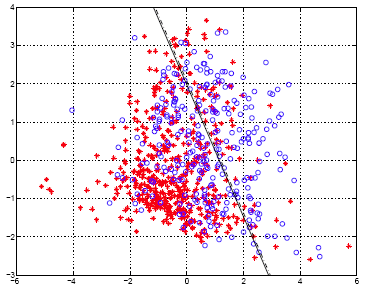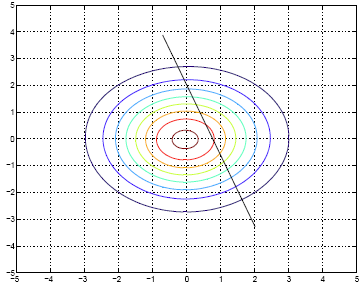# 9.2.6 - Example - Diabetes Data SetPrinter-friendly version

Let's take a look at specific data set. This is the diabetes data set from the UC Irvine Machine Learning Repository. It is a fairly small data set by today's standards. The original data had eight variable dimensions.  To simplify the example, we obtain the two prominent principal components from these eight variables. Instead of using the original eight dimensions we will just use these two principal components for this example.

The Diabetes data set has two types of samples in it. One sample type are healthy individuals and the other are individuals with a higher risk of diabetes. Here are the prior probabilities estimated for both of the sample types, first for the healthy individuals and second for those individuals at risk:

$\hat{\pi}_0 =0.651, \hat{\pi}_1 =0.349$

The first type has a prior probability estimated at 0.651. This means that for this data set about 65% of these belong to class 0 and the other 35% belong to class 1. Next, we computed the mean vector for the two classes separately:

$\hat{\mu}_0 =(-0.4038, -0.1937)^T, \hat{\mu}_1 =(0.7533, 0.3613)^T$

Then we computed $\hat{\Sigma}$ using the formulas discussed earlier.

$\hat{\Sigma}= \begin{pmatrix} 1.7949 & -0.1463\\ -0.1463 & 1.6656 \end{pmatrix}$

Once we have done all of this, we compute the linear discriminant function and find the classification rule.

Classification rule:

\begin{align*}\hat{G}(x) &=\begin{cases} 0 & 0.7748-0.6767x_1-0.3926x_2 \ge 0 \\ 1 & otherwise \end{cases} \\ & = \begin{cases} 0 & x_2 \le (0.7748/0.3926) - (0.6767/0.3926)x_1 \\ 1 & otherwise \end{cases} \end{align*}

In the first specification of the classification rule, plug a given x into the above linear function. If the result is greater than or equal to zero, then claim that it is in class 0, otherwise claim that it is in class 1.

Below is a scatter plot of the two principle components. The two classes are represented, the first, without diabetes, are the red stars (class 0), and the second class with diabetes are the blue circles (class 1). The solid line represents the classification boundary obtained by LDA. It seems as though the two classes are not that well separated. The dashed or dotted line is the boundary obtained by linear regression of an indicator matrix. In this case the results of the two different linear boundaries are very close.It is always a good practice to plot things so that if something went terribly wrong it would show up in the plots.

• Within training data classification error rate: 28.26%.
• Sensitivity: 45.90%.
• Specificity: 85.60%.

Here is the contour plot for the density for class 0. [Actually, the figure looks a little off - it should be centered slightly to the left and below the origin.] The contour plot for the density for class 1 would be similar except centered above and to the right.